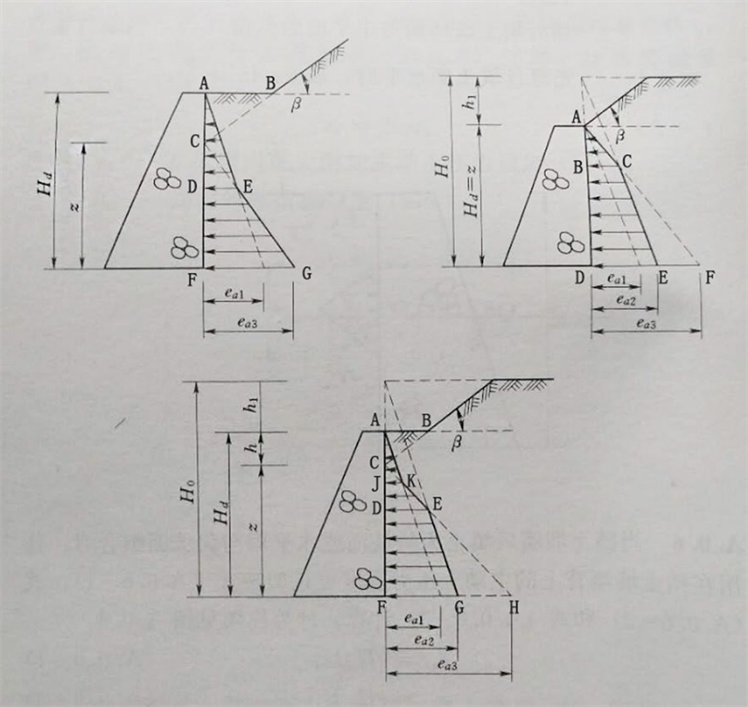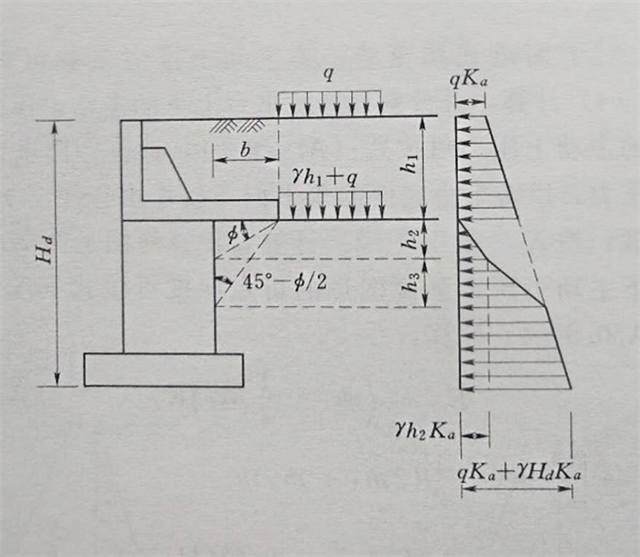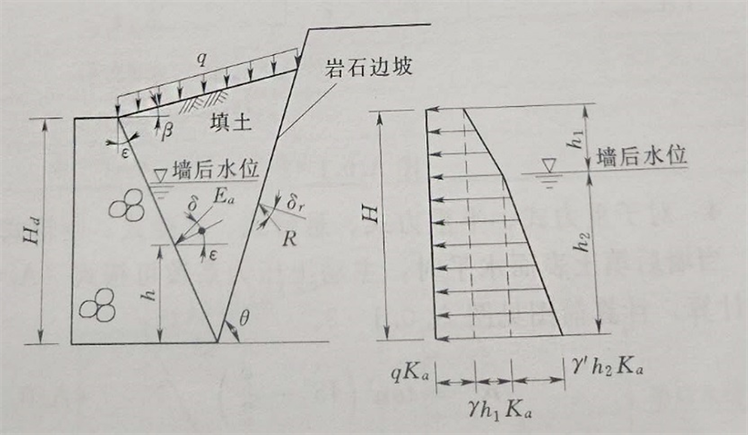﻿ 关于《水工挡土墙设计规范》(SL379-2007)有关土压力计算的商榷

# 关于《水工挡土墙设计规范》(SL379-2007)有关土压力计算的商榷Discussion on Earth Pressure Calculation in Hydraulic Retaining Wall Design Code (SL379-2007)

Abstract: The problems that active earth pressure coefficient and calculation formula of earth pressure are imperfection, incompleteness or error in hydraulic retaining wall design code (SL379-2007) are analyzed comprehensively and the formula of active Earth pressure is derived in 3 different cases when the back wall is composed by horizontal plane and infinite slope, the back wall is composed by finite slope and the back wall is composed by horizontal plane and finite slope to simplify the calculation. The calculation formula of the active earth pressure on the counterweight retaining wall is in contradiction with the calculation diagram and there are some calculation errors so we give out the right one. The influence factors of rock slope on earth pressure are discussed and the critical rupture angle formula and the discriminant model is given which can be used to guide the engineering design.

1. 前言

《水工挡土墙设计规范》 (SL379-2007)  附录A给出了各种情况下主动土压力系数、土压力计算图。笔者发现存在许多问题，有的是主动土压力系数计算不合理，有的是土压力计算公式不完善、不完整，有的是计算公式有缺陷，有的只给出土压力分布图，未给出具体计算式，在工程设计中，导致无法计算。

2. 挡土墙墙后填土为斜坡面、水平面与斜坡面组合时

2.1. 《水工挡土墙设计规范》计算模式

2.2. 主动土压力系数

${e}_{a1}=\gamma {H}_{d}{K}_{a1}$ (1)

${e}_{a2}=\gamma {H}_{0}{K}_{a2}$ (2)

${e}_{a3}=\gamma z{K}_{a3}$ (3)

2.2.1. 填土面为水平时的主动土压力系数

${K}_{a1}={\mathrm{tan}}^{2}\left({45}^{\circ }-\varphi /2\right)$ (4)Figure 1. Calculation chart of active earth pressure when the backfill is slope or the combination of horizontal and slope

2.2.2. 填土面坡角为 $\beta$ 时的主动土压力系数

${K}_{a3}=\frac{{\mathrm{cos}}^{2}\left(\varphi -\epsilon \right)}{{\mathrm{cos}}^{2}\epsilon \mathrm{cos}\left(\epsilon +\delta \right){\left[1+\sqrt{\frac{\mathrm{sin}\left(\varphi +\delta \right)\mathrm{sin}\left(\varphi -\beta \right)}{\mathrm{cos}\left(\epsilon +\delta \right)\mathrm{cos}\left(\epsilon -\beta \right)}}\right]}^{2}}$ (5)

2.3. 主动土压力大小

(图1(a))中主动土压力分布图面积大小就是主动土压力大小，但规范中没有给出具体计算公式，下面给出主动土压力计算公式。

2.3.1. 墙后为水平面与无限斜坡面组合时的土压力大小(图1a)

$c=\frac{{K}_{a1}}{{K}_{a3}-{K}_{a1}}h$ (6)

${E}_{a}=\frac{1}{2}\gamma {\left(h+c\right)}^{2}{K}_{a1}+\frac{1}{2}\gamma \left[{\left({H}_{d}-h\right)}^{2}-{c}^{2}\right]{K}_{a3}$ (7)

2.3.2. 墙后填土为有限斜坡面时的土压力(图1b)

$AB=d$，C点的位置为两条土压力线交点，由土压力强度相等 $\gamma \left({h}_{1}+d\right){K}_{a2}=\gamma d{K}_{a3}$ 解得：

$d=\frac{{K}_{a2}}{{K}_{a3}-{K}_{a2}}{h}_{1}$ (8)

${E}_{a}=\frac{1}{2}\gamma {H}_{d}^{2}{K}_{a3}-\frac{1}{2}\gamma \left({H}_{d}{K}_{a3}-{H}_{0}{K}_{a2}\right)\left({H}_{d}-d\right)$ (9)

2.3.3. 墙后填土为水平面与有限斜坡面组合时的土压力(图1c)

$c=\frac{{K}_{a1}}{{K}_{a3}-{K}_{a1}}h$ (10)

$d=\frac{{K}_{a2}}{{K}_{a3}-{K}_{a2}}\left(h+{h}_{1}\right)$ (11)

${E}_{a}=\frac{1}{2}\gamma {\left(h+c\right)}^{2}{K}_{a1}+\frac{1}{2}\left[\left(\gamma \left(h+c\right){K}_{a1}+\gamma d{K}_{a3}\right)\left(d-c\right)\right]+\frac{1}{2}\left(\gamma d{K}_{a3}+\gamma {H}_{0}{K}_{a2}\right)\left({H}_{d}-h-d\right)$ (12)

3. 衡重式挡土墙土压力

3.1. 《水工挡土墙设计规范》计算模式Figure 2. Calculation chart of active earth pressure for counterweight retaining wall

3.2. 主动土压力系数

3.3. 主动土压力分布及大小

${p}_{a}=q{K}_{a}+\gamma z{K}_{a}$ (13)

$z={h}_{1}$${p}_{a}=q{K}_{a}+\gamma {h}_{1}{K}_{a}$$z={H}_{d}$${p}_{a}=q{K}_{a}+\gamma {H}_{d}{K}_{a}$，即为墙底主动土压力强度； $z={h}_{1}+{h}_{2}+{h}_{3}$${p}_{a}=q{K}_{a}+\gamma \left({h}_{1}+{h}_{2}+{h}_{3}\right){K}_{a}$，分布图见图2 (包括图中空白的面积在内的梯形面积)。

3.3.1. 第一种情况

${h}_{3}$ 底端水平线与斜线不相交，交点处的土压力强度不相等，设置衡重台计算的 ${p}_{a}=\gamma {h}_{2}{K}_{a}+\gamma {h}_{3}{K}_{a}$，不受减压平台影响计算的 ${p}_{a}=q{K}_{a}+\gamma \left({h}_{1}+{h}_{2}+{h}_{3}\right){K}_{a}$，需要重新绘制主动土压力强度分布图，计算土压力大小。

3.3.2. 第二种情况

${h}_{3}$ 底端水平线与斜线相交，交点处的土压力强度只能有一个，即不受减压平台影响计算值 ${p}_{a}=q{K}_{a}+\gamma \left({h}_{1}+{h}_{2}+{h}_{3}\right){K}_{a}$，主动土压力强度分布图不变，但衡重式挡土墙衡重台下的主动土压力计算公式(A.0.8-2)有误，正确表达式为 ：

${E}_{a2}=\left[\frac{1}{2}\gamma {h}_{2}^{2}+\frac{1}{2}\left(q+\gamma {h}_{1}+2\gamma {h}_{2}+\gamma {h}_{3}\right){h}_{3}+\frac{1}{2}\left(2q+\gamma \left({h}_{1}+{h}_{2}+{h}_{3}\right)+\gamma {H}_{d}\right)\left({H}_{d}-{h}_{1}-{h}_{2}-{h}_{3}\right)\right]{K}_{a}$ (14)

4. 挡土墙墙后有较陡峻的稳定岩石坡面时的土压力

4.1. 有限范围填土的判别条件Figure 3. Calculation chart of earth pressure when there is a steep and stable rock face behind the wall

$\mathrm{tan}{\theta }_{cr}=\frac{\mathrm{sin}\beta \cdot {s}_{q}+\mathrm{cos}\left(\epsilon +\varphi +\delta \right)}{\mathrm{cos}\beta \cdot {s}_{q}-\mathrm{sin}\left(\epsilon +\varphi +\delta \right)}$ (15)

${s}_{q}=\sqrt{\frac{\mathrm{cos}\left(\epsilon +\delta \right)\mathrm{sin}\left(\varphi +\delta \right)}{\mathrm{cos}\left(\epsilon -\beta \right)\mathrm{sin}\left(\varphi -\beta \right)}}$ (16)

4.2. 主动土压力系数

$\theta >{\theta }_{cr}$

${K}_{a}=\frac{\mathrm{cos}\left(\epsilon -\beta \right)\mathrm{cos}\left(\theta -\epsilon \right)\mathrm{sin}\left(\theta -{\delta }_{R}\right)}{{\mathrm{cos}}^{2}\epsilon \mathrm{cos}\left(\theta -\epsilon -\delta -{\delta }_{R}\right)\mathrm{sin}\left(\theta -\beta \right)}$ (17)

$\theta <{\theta }_{cr}$

${K}_{a}=\frac{{\mathrm{cos}}^{2}\left(\varphi -\epsilon \right)}{{\mathrm{cos}}^{2}\epsilon \mathrm{cos}\left(\epsilon +\delta \right){\left[1+\sqrt{\frac{\mathrm{sin}\left(\varphi +\delta \right)\mathrm{sin}\left(\varphi -\beta \right)}{\mathrm{cos}\left(\delta +\epsilon \right)\mathrm{cos}\left(\epsilon -\beta \right)}}\right]}^{2}}$ (18)

4.3. 主动土压力

${E}_{a}=qH{K}_{a}+\frac{1}{2}\gamma {h}_{1}^{2}{K}_{a}+\gamma {h}_{1}{h}_{2}{K}_{a}+\frac{1}{2}{\gamma }^{\prime }{h}_{2}^{2}{K}_{a}$ (19)

5. 结论

1) 规范附录中式(附A.0.6-1)~(附A.0.6-3)中的主动土压力系数采用了同一表达符号，显然不妥，应根据实际情况采用不同的主动土压力系数。

2) 墙后为水平面与无限斜坡面组合、填土为有限斜坡面、填土为水平面与有限斜坡面组合等情况下的土压力分别按式(7) (9) (12)计算。

3) 衡重台下的主动土压力计算公式(附A.0.8-2)有误，计算公式与计算简图(附Ａ.0.8)相互矛盾，正确计算公式按(14)计算。

4) 朗肯土压力的破裂角 ${\theta }_{cr}$$\left({45}^{\circ }+\frac{\varphi }{2}\right)$ ；库仑土压力的破裂角 ${\theta }_{cr}$ 按(15)~(16)计算。

 中华人民共和国水利行业标准. 水工挡土墙设计规范(SL379-2007) [S]. 北京.

 刘福臣, 等. 工程地质与土力学[M]. 第三版. 郑州: 黄河水利出版社, 2020.

 刘福臣, 林世乐. 带减压平台挡土结构的土压力计算[J]. 港工技术, 2008(4): 41-42.

 杨学强, 等. 对挡土墙上主动土压力的再研究[J]. 力学与实践, 1999(3): 37-41.

 刘福臣, 等. 有限范围填土土压力计算模式[J]. 工程勘察, 2007(8):8-10..

Top